# What is a Solar PV Module?

A single solar cell cannot provide required useful output. So to increase output power level of a PV system, it is required to connect number of such PV solar cells. A solar module is normally series connected sufficient number of solar cells to provide required standard output voltage and power. One solar module can be rated from 3 watts to 300 watts. The solar modules or PV modules are commercially available basic building block of a solar electric power generation system.
Actually a single solar PV cell generates very tiny amount that is around 0.1 watt to 2 watts. But it is not practical to use such low power unit as building block of a system. So required number of such cells are combined together to form a practical commercially available solar unit which is known as solar module or PV module.

In a solar module the solar cells are connected in same fashion as the battery cell units in a battery bank system. That means positive terminals of one cell connected to negative terminal voltage of solar module is simple sum of the voltage of individual cells connected in series in the module.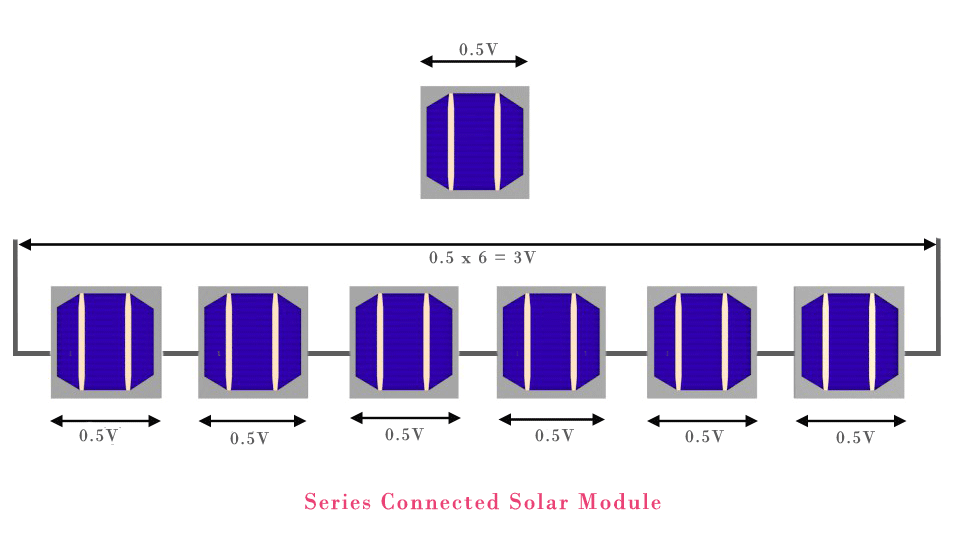The normal output voltage of a solar cell is approximately 0.5 V hence if 6 such cells are connected in series then the output voltage of the cell would be 0.5 × 6 = 3 Volt.

## Ratings of Solar Module

The output from a solar module depends upon some conditions such as ambient temperature and intensity of incidence light. Hence the rating of a solar module must be specified under such conditions. It is standardized practice to express rating of PV or solar module at 25oC temperature and 1000 w/m2 light radiation. The solar modules are rated with their output open circuit voltage (Voc), short circuit current (Isc) and peak power (Wp).

That means these three parameters (Voc, Isc and Wp) can be delivered by a solar module safely at 25oC and 1000 w/m2 solar radiations.
These conditions i.e. 25oC temperature and 1000 w/m2 solar radiations are collectively called Standard Test Conditions.
The Standard Test Conditions may not be available at site where the solar modules to be installed. This is because the solar radiations and temperature vary with location and time.

## V-I Characteristic of Solar Module

If we draw a graph by taking X-axis as voltage axis and Y-axis as currents of a solar module, then the graph will represent V-I characteristic of a solar module.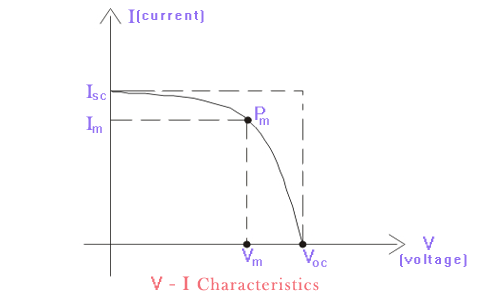## Short Circuit Current of PV Module

Under Standard Test Condition positive and negative terminal of a solar module are short circuited, then the current delivered by the module is short circuit current. Bigger value of this current indicates betterness of the module.
Although under standard test condition, this current also depends upon the area of the module exposed to the light. As it depends upon area, it is better to express by short circuit current per unit area.
This is deneted as Jsc.
Hence,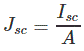Where, A is the area of the module exposed to the standard light radiation (1000w/m2). Short circuit current of a pv module also depends upon solar cell manufacturing technology.

## Open Circuit Voltage (Voc)

The voltage output of a solar module under standard test condition, when the terminals of the modules are not connected with any load. This rating of solar module mainly depends upon the technology used to manufacture solar cells of the module. More Voc indicates betterness of the solar module. This open circuit voltage of a solar module also depends upon operating temperature.

## Maximum Power Point

This is the maximum amount of power which can deliver by the module Under Standard Test Conditions. For a fixed dimension of a module higher the maximum power better the module. Maximum power also called peak power and this is denoted as Wm or Wp.
A solar module can be operated in any voltage and current combination upto Voc and Isc.
But for a particular current and voltage combination under standard conditions the output power is maximum. If we proceed through y-axis of the V-I characteristic of a solar module, we will find the power output increases nearly linearly with current but after a certain current power output will fall down as it approaches to the short circuit current as at short circuit condition the voltage is considered to be ideally zero across the terminals of the solar module. So it is clear that maximum output power of a solar module does not occur at maximum current i.e. short circuit current instead it occurs at certain current which is less than short circuit current (Isc). This current at which maximum output power occurs is denoted as Im.
Similarly maximum power of a solar cell does not occur at open circuit voltage as it is open circuit condition and current through the cell is considered to be ideally zero, at this condition. But similarly as previous case, maximum power in a solar module occurs at a voltage lower than open circuit voltage (Voc). The voltage at which maximum power output occurs is denoted as Vm. The maximum power of a solar module is given as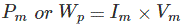The current and voltage at which maximum power occurs are referred as, current and voltage at maximum power point respectively.

## Fill Factor of a Solar Module

Fill factor of a solar module is defined as the ratio of maximum power (Pm = Vm x Im) to product of open circuit voltage (Voc) and short circuit current (Isc).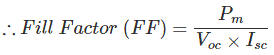Higher the Fill Factor(FF), better is the solar module.

## Efficiency of Solar Module

Efficiency of solar module is defined as the ratio of maximum power at standard test condition, to the input power. Input power of a solar module is solar radiation which is considered as 1000 w/m2. So, actual input power to the cell is 1000A W. Where, A is the exposed area of the solar module.
Therefore, efficiency,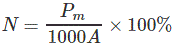## Number of Cells in Module

Number of cells in a module depends upon the standard voltage requirement per module. In 1980’s solar modules were mainly manufactured for charging 12 Volt batteries. But for charging a 12 Volt battery it is required to have sufficiently higher output voltage of the module than 12 Volt. It was standard practice to design a solar module with maximum voltage rating (Vm) of 15 Volt. This module of 15 Volt becomes standard module from those days.
The number of solar cells to be connected in series to achieve standard voltage output depends upon the open-circuit voltage (Voc) of the individual cells.
The Voc of a solar cell depends upon mainly its manufacturing techniques. The table below shows the open-circuit voltage of different solar cells at standard test conditions.

 Solar Cell Types Open Circuit Voltage at STC Mono Crystalline Silicon Solar Cell 0.55 to 0.68 V Poly Crystalline Silicon Solar Cell 0.55 to 0.65 V Amorphous Silicon Solar Cell 0.7 to 1.1 V Cadmium Telluride Solar Cell 0.8 to 1.0 V Copper Indium Gallium Selenide Solar Cell 0.5 to 0.7 V Gallium Indium Phosphide/ Gallium Arsenide / Gallium Solar Cell 1 to 2.5 V

For a crystalline solar cell the open-circuit voltage, is about 0.5 V, as shown in the table above. The voltage Voc is mentioned at 25oC but at the temperature higher than 25oC the value of this voltage drops nearly by 0.08 V.
So at normal operating temperature the voltage available across the terminals of each crystalline solar cell is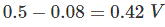Now, it is standard to make a solar module which can give 15 V open-circuit voltage at any condition.
Hence, the required number of solar cells to construct such solar module is,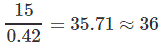So, 36 numbers of crystalline solar cells are required to build a standard solar module of 15 V.

Want To Learn Faster? 🎓
Get electrical articles delivered to your inbox every week.
No credit card required—it’s 100% free.